﻿ 基于堆栈降噪自编码器和LBP的人脸识别算法

# 基于堆栈降噪自编码器和LBP的人脸识别算法Face Recognition Algorithm Based on Stack Denoising Autoencoders and LBP

Abstract: In view of the weak robustness of the traditional face recognition algorithm, the low accuracy of classification and the slow operation rate, this paper proposes a new LBP + SDAE facial expression recognition algorithm, which is based on Local Binary Pattern and Stack Denoising Autoencoders. First, the image is processed by histogram equalization and the feature is extracted with LBP. Then, the scale normalization is followed by using SDA to extract the feature again, to reduce image denoising and dimensionality. Finally, the SVM algorithm was selected as classifier for the recognition of images. This method not only improves the accuracy of classification, but also accelerates the computation speed. The YALE face database was used to test the proposed method. The experiment results show that it has higher accuracy and robustness compared with the traditional face recognition algorithm.

1. 引言

2. 局部二值模式

2.1. 原始LBP算子

LBP的算法思想就是利用局部的结构提取窗口特征，再利用统计直方图取得整体特征。如图1所示，LBP是定义在一个3 * 3的领域内，以中心像素的灰度值为阈值，与其相邻的其余8个像素灰度值作比较，若相邻的像素灰度值比中心的像素灰度值大，则标记为1，否则为0。接着按顺序依次排列，转化成一个8位数的二进制数，相对应的十进制就是中心像素的LBP值。那么，这里的二进制数为01101001，对应的十进制数为105，也就是说这个中心像素的LBP值为105。这个LBP值就表示了图像的局部纹理特征。

2.2. 圆形LBP算子

3. 自编码器的基本模型

3.1. 自编码器

$Z=f\left({W}_{1}X+{b}_{1}\right)$ (1)

$Y=g\left({W}_{2}Z+{b}_{2}\right)$ (2)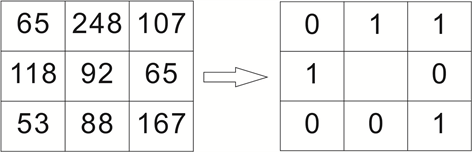Figure 1. A field of 3 * 3 in LBP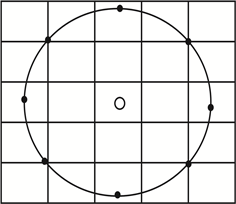LBP162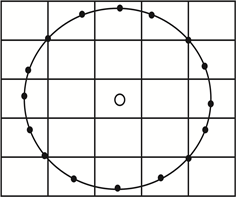LBP82

Figure 2. A circular field of LBP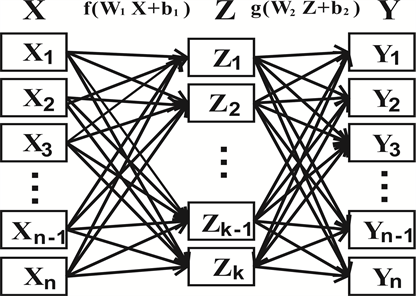Figure 3. An autoencoder with 1 layer of hidden layers

$L\left(x,y\right)=-{\sum }_{i=1}^{n}\left[{x}_{i}\mathrm{log}{y}_{i}+\left(1-{x}_{i}\right)\mathrm{log}\left(1-{y}_{i}\right)\right]$ (3)

$L\left(x,y\right)={‖x-y‖}^{2}$ (4)

$\mathrm{min}L\left(x,y\right)={‖x-y‖}^{2}$ (5)

$\mathrm{min}L\left(x,y\right)={‖x-y‖}^{2}+\frac{\lambda }{2}{‖W‖}^{2}$ (6)

3.2. 降噪自动编码器

$\mathrm{min}L\left(x,y\right)={‖x-y‖}^{2}$ (而不是 $\mathrm{min}L\left(\stackrel{¯}{x},y\right)={‖\stackrel{¯}{x}-y‖}^{2}$ ) (7)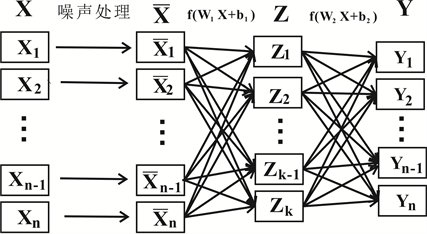Figure 4. Denoising autoencoder

$\stackrel{¯}{{x}_{i}}={x}_{i}+Normal\left(0,1\right)$ (8)

3.3. 堆栈自编码器

$\theta =\left\{{W}_{11},{b}_{11},{W}_{12},{b}_{12}\right\}$ (9)

4. 支持向量机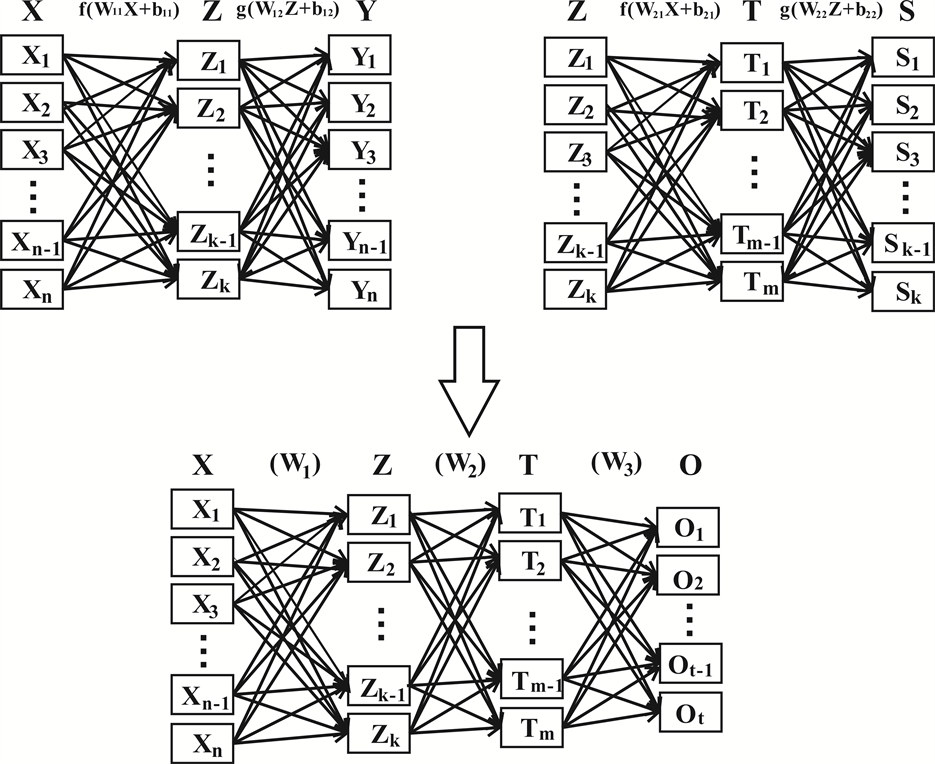Figure 5. Stack autoencoder

SVM的算法思想就是找到一个最优分类超平面，在满足分类要求和分类精度的条件下，使得训练样本和超平面的距离最大化。以两类数据分类为例，假定有训练样本集 $\left({x}_{1},{y}_{1}\right),\left({x}_{2},{y}_{2}\right),\cdots ,\left({x}_{n},{y}_{n}\right)$${x}_{i}\in {R}^{d}$${y}_{i}\in \left\{+1,-1\right\}$ ，其中每个样本是d维向量，y是类别标号， ${w}_{1}$ 类用+1表示， ${w}_{2}$ 类用-1表示。若这些样本是线性可分的，则存在超平面：

$g\left(x\right)=\left(w\cdot x\right)+b={w}^{\text{T}}+b=0$ (10)

${y}_{i}\left[\left(w\cdot {x}_{i}\right)+b\right]\ge 1,\text{\hspace{0.17em}}\text{\hspace{0.17em}}i=1,2,\cdots ,N$ (11)

${\mathrm{min}}_{w,b}{\mathrm{max}}_{\alpha }L\left(w,b,\alpha \right)=\frac{1}{2}\left(w\cdot w\right)-{\sum }_{i=1}^{N}{\alpha }_{i}\left\{{y}_{i}\left[\left(w\cdot {x}_{i}\right)+b\right]-1\right\}$ (12)

$f\left(x\right)=\mathrm{sgn}\left\{g\left(x\right)\right\}=\mathrm{sgn}\left\{\left({w}^{*}\cdot x\right)+b\right\}=\mathrm{sgn}\left\{{\sum }_{i=1}^{N}{\alpha }_{i}^{\ast }{y}_{i}\left({x}_{i}\cdot x\right)+{b}^{*}\right\}$ (13)

${y}_{i}\left[\left(w\cdot {x}_{i}\right)+b\right]-1\ge 0,\text{\hspace{0.17em}}\text{\hspace{0.17em}}i=1,2,\cdots ,N$ (14)

${y}_{i}\left[\left(w\cdot {x}_{i}\right)+b\right]-1+{\xi }_{i}\ge 0,\text{\hspace{0.17em}}\text{\hspace{0.17em}}i=1,2,\cdots ,N$ (15)

$\underset{w,b}{\mathrm{min}}\frac{1}{2}\left(w\cdot w\right)+C\left({\sum }_{i=1}^{N}{\xi }_{i}\right)$ (16)

$\begin{array}{l}{\mathrm{min}}_{w,b,{\xi }_{i}}{\mathrm{max}}_{\alpha }L\left(w,b,\alpha \right)\\ =\frac{1}{2}\left(w\cdot w\right)+C{\sum }_{i=1}^{N}{\xi }_{i}-{\sum }_{i=1}^{N}{\alpha }_{i}\left\{{y}_{i}\left[\left(w\cdot {x}_{i}\right)+b\right]-1+{\xi }_{i}\right\}-{\sum }_{i=1}^{N}{\gamma }_{i}{\xi }_{i}\end{array}$ (17)

$f\left(x\right)=\mathrm{sgn}\left\{g\left(x\right)\right\}=\mathrm{sgn}\left\{\left({w}^{*}\cdot x\right)+b\right\}=\mathrm{sgn}\left\{{\sum }_{i=1}^{N}{\alpha }_{i}^{\ast }{y}_{i}\left({x}_{i}\cdot x\right)+{b}^{*}\right\}$ (18)

Sigmoid函数： $K\left(x,{x}^{\prime }\right)=\mathrm{tanh}\left(v\left(x\cdot {x}^{\prime }\right)+c\right)$ ，采用这种核函数的SVM实现的是在 和 满足一定取值条件下等价与包含一个隐层的多层感知机神经网络。

5. 仿真和算法过程

5.1. 在Yale Face Database上的实验

5.2. 算法过程

5.2.1. 图像预处理

5.2.2. 栈式降噪自编码器提取人脸特征

5.2.3. 构建多类SVM及核函数的选择

5.2.4. 实验结果

5.3. 实验分析

1) 用LBP算法，可以去除光照和角度的影响，增强了图像的特征，提高了识别率；

2) 使用SDA算法，可以快速降维，把特征向量从32,256维降到16维，这样提高了运算率和识别率。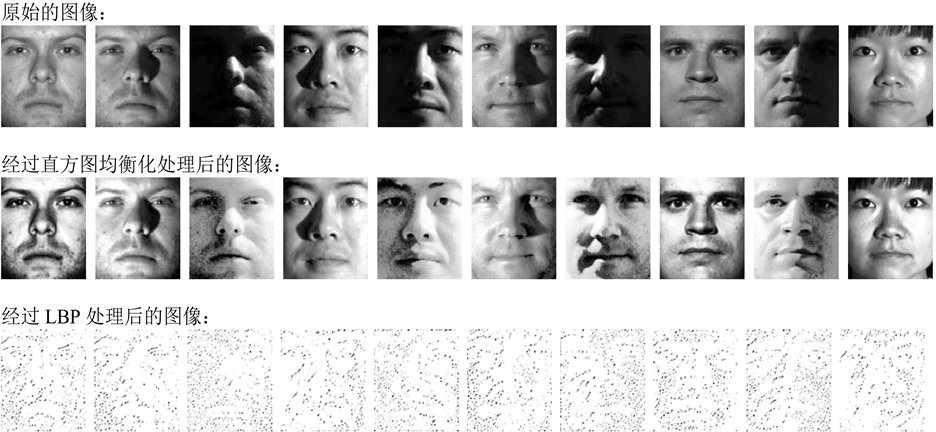Figure 5. Face contrast after preprocessingTable 1. Comparison of the recognition rate of the model

6. 结论

 Yu, Z., Liu, F., Liao, R.T., et al. (2018) Improvement of Face Recognition Algorithm Based on Neural Network. 2018 10th Interna-tional Conference on Measuring Technology and Mechatronics Automation (ICMTMA), Changsha, 10-11 February 2018.
https://doi.org/10.1109/ICMTMA.2018.00062

 Chen, A.P., Pan, L. Tong, Y.B. and Ning, N.. (2010) Face Detection Tech-nology Based on Skin Color Segmentation and Template Matching. IEEE 2nd International Workshop on Education Technology and Computer Science, Wuhan, 6-7 March 2010, 708-711.
https://doi.org/10.1109/ETCS.2010.465

 Tian, J. and Zheng, Y.Z. (2008) Application of Template Matching Technology in Image Recognition. Sensors and Microsystems, 27, 112-114.

 He, G. and Gan, J. (2004) A Method for Singular Value Feature Extraction of Face Image. Proceedings of 2004 International Symposium on Intel-ligent Multimedia, Video and Speech Processing, Hong Kong, 20-22 October 2005, 37-40.
https://doi.org/10.1109/ISIMP.2004.1433994

 Lu, Y. and Tian, Q. (2009) Discriminant Subspace Analysis: An Adaptive Approach for Image Classification. IEEE Transactions on Multimedia, 11, 1289-1300.
https://doi.org/10.1109/TMM.2009.2030632

 Siwek, K. and Osowski, S. (2017) Autoencoder versus PCA in Face Recogni-tion. 2017 18th International Conference on Computational Problems of Electrical Engineering (CPEE), Kutna Hora, 11-13 September 2017, 1-4.
https://doi.org/10.1109/CPEE.2017.8093043

 Senthilkumar, R. and Gnanamurthy, R.K. (2017) Performance Improvement in Classification Rate of Appearance Based Statistical Face Recognition Methods Using SVM Classifier. 2017 4th International Confer-ence on Advanced Computing and Communication Systems (ICACCS), Coimbatore, 6-7 January 2017, 1-7.
https://doi.org/10.1109/ICACCS.2017.8014584

 Sun, Z.P. and Sun, J.G. (2010) Face Recognition Based on Fractal and Hidden Markov Model. 2010 3rd International Symposium on Electronic Commerce and Security (ISECS), Guangzhou, 29-31 July 2010.
https://doi.org/10.1109/ISECS.2010.71

 Kumar, V., Kalitin, D. and Tiwari, P. (2017) Unsupervised Learning Dimensionality Reduction Algorithm PCA for Face Recognition. 2017 International Conference on. Computing, Communication and Automation (ICCCA), Greater Noida, 5-6 May 2017.
https://doi.org/10.1109/CCAA.2017.8229826

 Lecun, Y., Bengio, Y. and Hinton, G. (2015) Deep Learning. Nature, 521, 436-444.
https://doi.org/10.1038/nature14539

 Sujay, S.H.S., Reddy, M. and Ravi, J. (2017) Face Recognition Using Extended LBP Features and Multilevel SVM Classifier. 2017 International Conference on Electrical, Electronics, Communication, Computer, and Optimization Techniques (ICEECCOT), Mysuru, 15-16 December 2017.
https://doi.org/10.1109/ICEECCOT.2017.8284596

 Xu, Z. and Fu, T. (2017) A Modulation Classification Method in Cogni-tive Radios System Using Stacked Denoising Sparse Autoencoder. IEEE Radio and Wireless Symposium (RWS), Phoenix, AZ, 15-18 January 2017, 218-220.

 Jiang, L., Ge, Z. and Song, Z. (2017) Semi-Supervised Fault Classification Based on Dynamic Sparse Stacked Auto-Encoders Model. Chemometrics & Intelligent Laboratory Systems, 168, 72-83.
https://doi.org/10.1016/j.chemolab.2017.06.010

 Zhang, S., Wang, J., Tao, X., et al. (2016) Constructing Deep Sparse Cod-ing Network for Image Classification. Pattern Recognition, 64, 130-140.
https://doi.org/10.1016/j.patcog.2016.10.032

 Ejaz, U.H., Xu, H.R. and Muhammad, I. (2017) Face Recognition by SVM Using Local Binary Patterns. IEEE Web Information Systems and Applications Conference (WISA), Liuzhou, 11-12 November 2017, 172-175.

Top